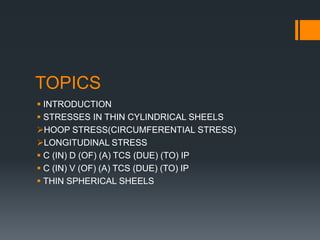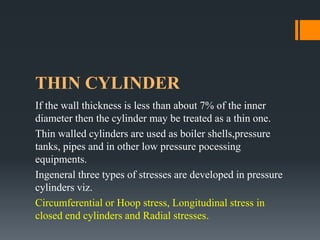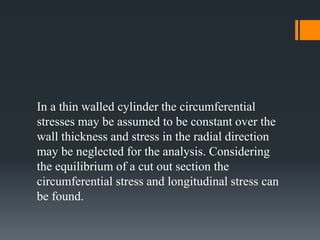Se está descargando tu SlideShare. ×

# Thin cylinders 1Cargando en…3
×

1 de 30
1 de 30

# Thin cylinders 1

Thin cylinders

Thin cylinders

### Thin cylinders 1

1. 1. TOPICS  INTRODUCTION  STRESSES IN THIN CYLINDRICAL SHEELS HOOP STRESS(CIRCUMFERENTIAL STRESS) LONGITUDINAL STRESS  C (IN) D (OF) (A) TCS (DUE) (TO) IP  C (IN) V (OF) (A) TCS (DUE) (TO) IP  THIN SPHERICAL SHEELS
2. 2. THIN CYLINDER If the wall thickness is less than about 7% of the inner diameter then the cylinder may be treated as a thin one. Thin walled cylinders are used as boiler shells,pressure tanks, pipes and in other low pressure pocessing equipments. Ingeneral three types of stresses are developed in pressure cylinders viz. Circumferential or Hoop stress, Longitudinal stress in closed end cylinders and Radial stresses.
3. 3. In a thin walled cylinder the circumferential stresses may be assumed to be constant over the wall thickness and stress in the radial direction may be neglected for the analysis. Considering the equilibrium of a cut out section the circumferential stress and longitudinal stress can be found.
4. 4. 2. Longitudinal Stress (σL ) – This stress is directed along the length of the cylinder. This is also tensile in nature and tends to increase the length. 3. Radial pressure ( pr ) – It is compressive in nature. Its magnitude is equal to fluid pressure on the inside wall and zero on the outer wall if it is open to atmosphere. 1. Hoop or Circumferential Stress (σC) – This is directed along the tangent to the circumference and tensile in nature. Thus, there will be increase in diameter.
5. 5. σ C σ L 1. Hoop Stress (C) 2. Longitudinal Stress (L) 3. Radial Stress (pr) Element on the cylinder wall subjected to these three stresses σ C σ C σC p σ L σ L σ L p p pr σ Lσ L σ C σ C pr pr
6. 6. INTRODUCTION: A cylinder or spherical shell is considered to be thin when the metal thickness is small compared to internal diameter. i. e., when the wall thickness, ‘t’ is equal to or less than ‘d/10 to d/15’, where ‘d’ is the internal diameter of the cylinder or shell, we consider the cylinder or shell to be thin, otherwise thick. In thin cylindrical stress hoop stress and longitudinal stresses are constant over the thickness and radial stresses are negligible. Magnitude of radial pressure is very small compared to other two stresses in case of thin cylinders and hence neglected. THIN CYLINDERS
7. 7. Longitudinal axisLongitudinal stress Circumferential stress t The stress acting along the circumference of the cylinder is called circumferential stresses whereas the stress acting along the length of the cylinder (i.e., in the longitudinal direction ) is known as longitudinal stress
8. 8. The bursting will take place if the force due to internal (fluid) pressure (acting vertically upwards and downwards) is more than the resisting force due to circumferential stress set up in the material. p σc σc P - internal pressure (stress) σc –circumferential stress
9. 9. P - internal pressure (stress) σc – circumferential stress dL σc p t
10. 10. EVALUATION OF CIRCUMFERENTIAL or HOOP STRESS (σC): Consider a thin cylinder closed at both ends and subjected to internal pressure ‘p’ as shown in the figure. Let d=Internal diameter, t = Thickness of the wall L = Length of the cylinder. p d t σcσc dlt p d
11. 11. To determine the Bursting force across the diameter: Consider a small length ‘dl’ of the cylinder and an elementary area ‘dA’ as shown in the figure. rpp dθdldAdF  dθdldFx  θcos 2 d p dA σcσc dlt p d dθ θ Force on the elementary area, Horizontal component of this force dθdl  2 d p dθdldFy  θsin 2 d p Vertical component of this force
12. 12. The horizontal components cancel out when integrated over semi-circular portion as there will be another equal and opposite horizontal component on the other side of the vertical axis. sin 2 d pforceburstingldiametricaTotal 0     dθdl dA σcσc dlt p θ d dθ   surface.curvedtheofareaprojectedp dpcosdl 2 d p 0   dl 
13. 13. dlcc  tσ2)σstressntialcircumfereto(dueforceResisting dldl  dptσ2i.e., c dL σc p t forceBurstingforceResistingum,equillibriUnder  Circumferential stress 𝜎𝑐 = 𝑝𝑑 2𝑡
14. 14. LONGITUDINAL STRESS (σL): p σL The force, due to pressure of the fluid, acting at the ends of the thin cylinder, tends to burst the cylinder as shown in figure P A B The bursting of the cylinder takes place along the section AB
15. 15. EVALUATION OF LONGITUDINAL STRESS (σL): d 4 π pcylinder)ofend(on theforceburstingalLongitudin 2  p t σL tdπσforceResisting L  tdπforcethisresistingsectioncrossofArea  cylinder.theofmaterialtheofstressalLongitudinσLet L 
16. 16. tdπσd 4 π pi.e., L 2  UNDER EQULIBIRIUM BURSTING FORCE = RESISTING FORCE ∴ 𝑙𝑜𝑛𝑔𝑖𝑡𝑢𝑑𝑖𝑛𝑎𝑙 𝑠𝑡𝑟𝑒𝑠𝑠 𝜎𝐿 = 𝑝𝑑 4𝑡
17. 17. CHANGE IN DIMENSIONS OF A THIN CYLINDRICAL SHELL DUE TO INTERNAL PRESSURE
18. 18. Consider a thin cylindrical shell subjected to internal pressure p = internal pressure d = internal diameter of shell t = thickness of shell l = length of the shell WE KNOW THAT, σc = pd 2t and σL = pd 4t Let, δd= change in diameter of shell δl = change in length of shell ∴ circumferential strain, ε 1 = 𝛿𝑑 𝑑 = 𝜎 𝑐 𝐸 − 𝜎 𝑙 𝑚𝐸 = 𝑝𝑑 2𝑡𝐸 − 𝑝𝑑 4𝑡𝑚𝐸 ∈1= 𝑝𝑑 2𝑡𝐸 1 − 1 2𝑚
19. 19. ∈2= 𝛿𝑙 𝑙 = 𝜎𝑙 𝐸 − 𝜎𝑐 𝑚𝐸 = 𝑝𝑑 4𝑡𝐸 − 𝑝𝑑 2𝑡𝑚𝐸 ∈2= 𝑝𝑑 2𝑡𝐸 1 2 − 1 𝑚 ∴ 𝑙𝑜𝑛𝑔𝑖𝑡𝑢𝑑𝑖𝑛𝑎𝑙 𝑠𝑡𝑟𝑎𝑖𝑛 Change in diameter = 𝛿𝑑 =∈1 𝑑 Change in length = 𝛿𝑙 =∈2 𝑙 When the shell is subjected to an internal pressure, there will be an increase in the Diameter as well as the length of the shell
20. 20. CHANGE IN VOLUME OF A THIN CYLINDRICAL SHELL DUE TO INTERNAL PRESSURE
21. 21. Voloume of shell , 𝑉 = 𝜋 4 𝑑2 𝑙 ∴ Final volume v+𝛿𝑣 = 𝜋 4 (𝑑 + 𝛿𝑑)2 × 𝑙 + 𝛿𝑙 ∴ Change in voloume , 𝛿𝑣 = 𝑣 + 𝛿𝑣 − 𝑣 = 𝜋 4 (𝑑 + 𝛿𝑑)2 (𝑙 + 𝛿𝑙) − 𝜋 4 𝑑2 𝑙 = 𝜋 4 𝑑2 + 2. 𝛿𝑑. 𝑑𝑙 + 𝛿𝑑2 . 𝑙 + 𝑑2 . 𝛿𝑙 + 2𝛿𝑑. 𝑑. 𝛿𝑙 + 𝛿𝑑2 . 𝛿𝑙 − 𝜋 4 𝑑2 . 𝑙 = 𝜋 4 𝑑2. 𝛿𝑙 + 2𝛿𝑑. 𝑑. 𝑙 𝑛𝑒𝑔𝑙𝑒𝑐𝑡𝑖𝑛𝑔 𝑠𝑚𝑎𝑙𝑙 𝑞𝑢𝑎𝑛𝑡𝑖𝑡𝑖𝑒𝑠
22. 22. 𝛿𝑣 𝑉 = 𝜋 4 (𝑑2 . 𝛿𝑙 + 2. 𝛿𝑑. 𝑑. 𝑙) 𝜋 4 𝑑2 𝑙 = 𝛿𝑙 𝑙 + 2𝛿𝑑 𝑑 𝛿𝑣 𝑉 = 𝜖2 + 2. 𝜖1 ∴ 𝛿𝑣 = 𝑉(𝜀2 + 2. 𝜖1) ∵ 𝜖1 = 𝛿𝑑 𝑑 𝜀2 = 𝛿𝑙 𝑙 ∈1= 𝑐𝑖𝑟𝑐𝑢𝑚𝑓𝑒𝑟𝑒𝑛𝑡𝑖𝑎𝑙 𝑠𝑡𝑟𝑎𝑖𝑛 ∈2= 𝑙𝑜𝑛𝑔𝑖𝑡𝑢𝑑𝑖𝑛𝑎𝑙 𝑠𝑡𝑟𝑎𝑖𝑛
23. 23. THIN SPHERICAL SHELL
24. 24. Consider a thin spherical shell subjected to internal pressure p p= internal pressure d= internal dia of shell t= thickness of shell 𝜎 = stress in the shell material Total force, P= 𝜋 4 × 𝑑2 × 𝑝 Resisting section = 𝜋𝑑𝑡 ∴ Stress in the shell, 𝜎 = 𝑡𝑜𝑡𝑎𝑙 𝑓𝑜𝑟𝑐𝑒 𝑟𝑒𝑠𝑖𝑠𝑡𝑖𝑛𝑔 𝑠𝑒𝑐𝑡𝑖𝑜𝑛 Type equation here. = 𝜋 4 𝑑2×𝑝 𝜋𝑑𝑡 𝜎 = 𝑝𝑑 4𝑡
25. 25. CHANGE IN DIAMETER AND VOLUME OF A THIN SPHERICAL SHELL DUE TO INTERNAL PRESSURE
26. 26. Consider a thin spherical shell subjected to internal pressure d=internal dia of shell p=internal pressure t=thickness of the shell 𝜎= stress in the shell material We know that the thin spherical shell, 𝜎 = 𝑝𝑑 4𝑡 Strain in any direction, 𝜖 = 𝜎 𝐸 − 𝜎 𝑚𝐸 = 𝑝𝑑 4𝑡𝐸 − 𝑝𝑑 4𝑡.𝑚𝐸 = 𝑝𝑑 4𝑡𝐸 (1− 1 𝑚 )
27. 27. 2 4t pd 2t pd 2 σ-σ τstress,ShearMaximum other.eachto larperpendicuactandnormalarestressesthese Bothal.longitudinandntialCircumfereviz., point,anyatstressesprincipaltwoareThere :stressShearMaximum LC max    )5.(.................... 8t pd τi.e., max  σ C=(pd)/(2t) σC=(pd)/(2t) σ L=(pd)/(4t) σ L=(pd)/(4t)
28. 28. 2 4t pd 2t pd 2 σ-σ τstress,ShearMaximum :stressShearMaximum LC max    i.e. 𝜏 𝑚𝑎𝑥 = 𝑝𝑑 8𝑡